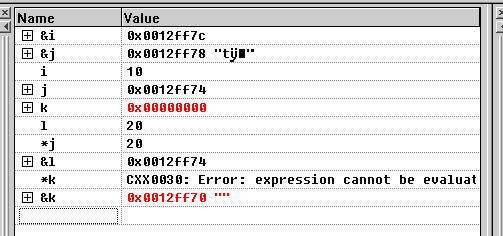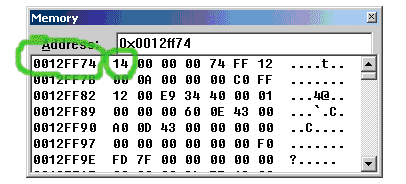MyProcess WebSharp Pacer建模

2009-01-20 作者：曹翼飞 来源：放飞技术网

 int main() { 　　int i=10; 　　int *j=&i; 　　if(!0) 　　{ 　　　　int l=20; 　　　　int *k=&l; 　　　　j=k; 　　　　k=0; 　　} 　　cout<<*j; 　　return 0; }

 int i=10;　 //i is 10 and &i is 0x0012ff7c int *j=&i;　//*j is 10 and &j is 0x0012ff7c 　　　　　　//显然可以看出此时两个变量指的是同一地址 if(!0) { 　　int l=20; //l is 20 and &l is 0x0012ff74 　　/*地址0x0012ff7c―0x0012ff75被占据。要说明的是， 　　这个数值很有可能因为电脑硬件的不同而不同。*/ 　　int *k=&l; //*k is 20 and &k is 0x0012ff74 　　//变量k与l指向同一地址。 　　j=k; 　//j is 0x0012ff74 and *j is 20 　　/*指针间的赋值，这个语句的意思是把k指向的地址负值给j。 　　此时这两个变量指向的是同一个地址，都是0x0012ff74，而那块地址存放的是20，所以也就有*j是20的原因。*/ } cout<<*j;　//*j is 20 and j is 0x0012ff74 /*此时同时可以看到k的地址是0x00000000，说明k这个变量已经被自动销毁，所以地址指零。但是j所指的并不是k，而是k所指的那段地址0x0012ff74，而由于此时j的生存周期还没有结束（j是在if意外定义的），所以j指向的这块地址并没有被收回，也就保存下来20这个数了。*/int * foo() { 　　int l=20; 　　return &l; } int main() { 　　int i=10; 　　int *j=&i; 　　j = foo(); 　　cout<<*j; 　　return 0; }

 void f1( int *& j) { 　　int l=20; 　　int *k=&l; 　　j=k; 　　k=0; } void any_function_use_local_variables() { 　　int a,b,c; 　　a=b=c=100; } int main() { 　　int i=10; 　　int *j=&i; 　　f1(j); 　　cout<<*j; 　　any_function_use_local_variables(); 　　cout<<*j; 　　return 0; }

 int main() { 　　int i=1; 　　while(0

 | 联系我们 | 招聘信息 | 火龙果软件 | 火龙果软件/UML软件工程组织致力于提高您的软件工程实践能力，我们不断地吸取业界的宝贵经验，向您提供经过数百家企业验证的有效的工程技术实践经验，同时关注最新的理论进展，帮助您“领跑您所在行业的软件世界”。
 资源网站: UML软件工程组织